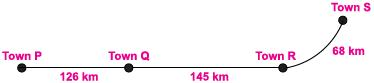# User ForumSubject :IMO    Class : Class 3

Garima travelled from Town S to Town Q and came back to Town S, and Latika travelled from Town R to Town P. Who travelled a longer distance and how much longer?(A) Latika, 155 km

(B) Garima, 145 km

(C) Garima, 155 km

(D) Latika, 145 km

## Ans 1: (Master Answer)

Class : Class 1

(C):

Distance travelled by Latika = (145 + 126)
= 271 km
Distance travelled by Garima = ( 68 + 145) x 2
= 213 x 2 = 426 km
Therefore, Garima travelled a longer distance .

Difference = 426 – 271 = 155 km

## Ans 2: (Master Answer)

Class : Class 1

(C):

Distance travelled by Latika = (145 + 126)
= 271 km
Distance travelled by Garima = ( 68 + 145) x 2
= 213 x 2 = 426 km
Therefore, Garima travelled a longer distance .

Difference = 426 – 271 = 155 km